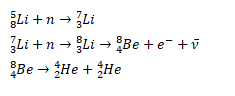# Lithium (Z=3) has two stable isotopes 6Li and 7Li.

Question:

Lithium ( $\mathrm{Z}=3)$ has two stable isotopes ${ }^{6} \mathrm{Li}$ and ${ }^{7} \mathrm{Li}$. When neutrons are bombarded on lithium sample, electrons and $\alpha$ particles are ejected. Write down the nuclear processes taking place.

Solution: